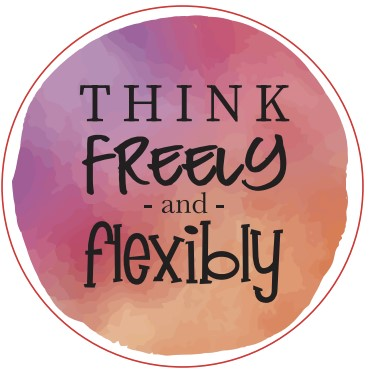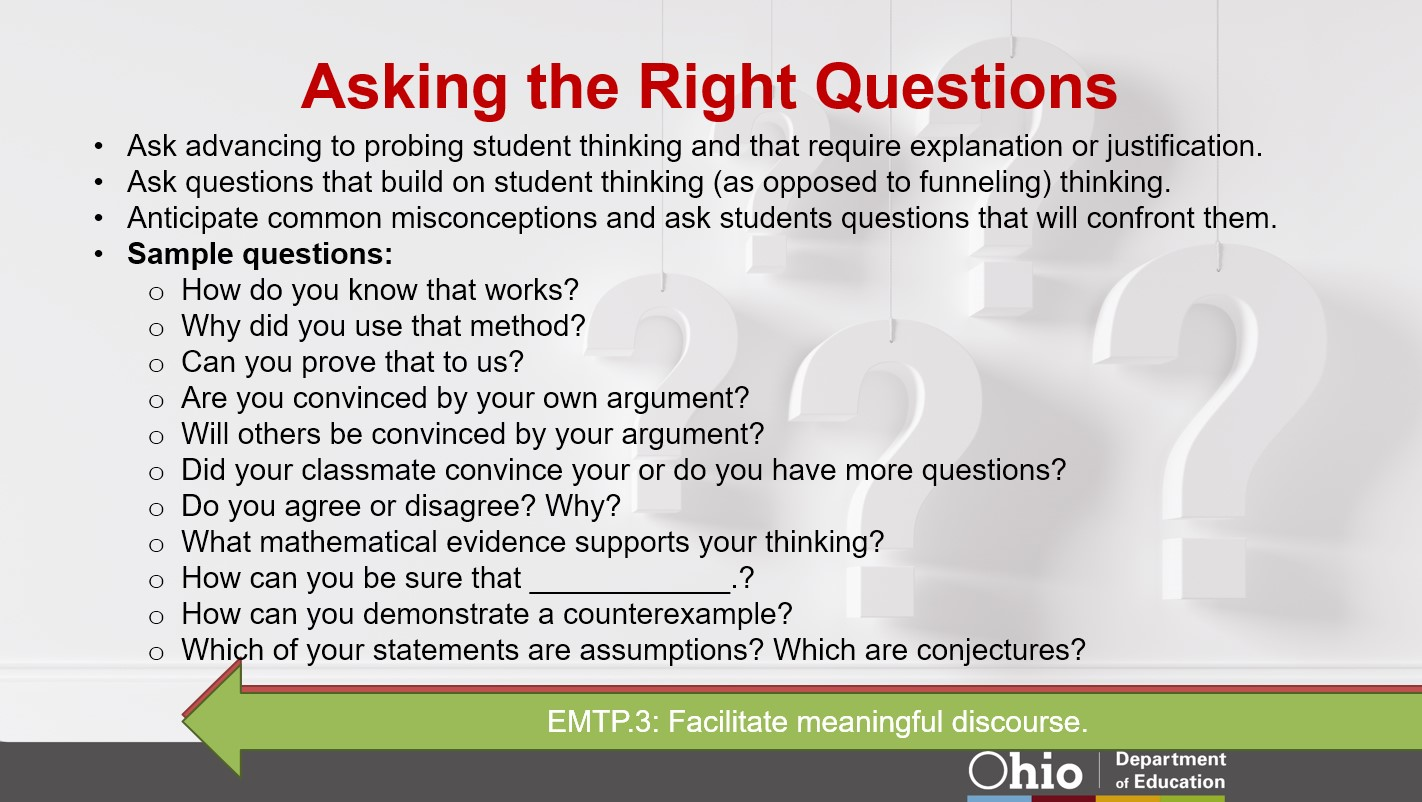# Mathematical Vocabulary Part 1

I often reference the 8 Standards of Mathematical Practice when I am working with teachers. Regardless of the set of standards your school or district utilizes, the vast majority also include the Standards for Mathematical Practice. Mathematical Practice Standards are separate from content standards. The graphic below gives us an easy to understand breakdown.The state of Ohio’s education site says, “The Standards for Mathematical Practice describe the skills that mathematics educators should seek to develop in their students.”

Per the site, CoreStandards.org , the Mathematical Practices are defined as,

“The Standards for Mathematical Practice describe varieties of expertise that mathematics educators at all levels should seek to develop in their students. These practices rest on important “processes and proficiencies” with longstanding importance in mathematics education. The first of these are the NCTM process standards of problem solving, reasoning and proof, communication, representation, and connections. The second are the strands of mathematical proficiency specified in the National Research Council’s report Adding It Up: adaptive reasoning, strategic competence, conceptual understanding (comprehension of mathematical concepts, operations and relations), procedural fluency (skill in carrying out procedures flexibly, accurately, efficiently and appropriately), and productive disposition (habitual inclination to see mathematics as sensible, useful, and worthwhile, coupled with a belief in diligence and one’s own efficacy).”

You may notice some familiar words. This is where my favorite thing to tell students comes from.We could spend a blog post on each of the 8 Practices, but many people already have. Some of my favorites are:

• Robert Kaplinsky : Robert attempts to rewrite the Mathematical Practices in a way that educators, parents, and students can understand them.
• Mike Flynn: Mike wrote a book to help educators gain a clear picture and to understand the 8 Standards of Mathematical Practice and how to implement them in a K-2 classroom. I’m sure what he has in this book can also be very helpful in a 3-6 classroom as well.
• Sarah VanDerwerf: Sarah is such an inspiration for those who follow her. She does such a great job of showing teachers in all grade bands things they can do in order to get students to acknowledge and believe they are mathematicians. This particular post always has me thinking about what I do with students and teachers.

Instead, I am going to focus on the vocabulary piece. Content standards are full of mathematical vocabulary and can often be confusing to both teachers and students. When do we use content-specific vocabulary? When do we use student-friendly vocabulary? How and when do we blend both together? When we talk about vocabulary we are asking our students to work within a few of the Mathematical Practices.

MP1: Make sense of problems and persevere in solving them.

MP2: Reason abstractly and quantitatively.

MP3: Construct viable arguments and critique the reasoning of others.

MP4: Model with mathematics.

MP6: Attend to precision.

Let’s break the Mathematical Practices down and how they relate to vocabulary.

MP1: Make sense of problems and persevere in solving them.

• Students need to be able to comfortably show their thinking in their work. Sometimes students will have to read or work through difficult problems. Students need to understand problems conceptually in order to apply their problem-solving strategies. Understanding and applying the proper vocabulary is critically important.
• When students make sense of problems, they are thinking contextually about problems, not procedurally.
• We need student dialogue and good questioning from the teacher to encourage “making sense” of the problem.

MP2: Reason abstractly and quantitatively.

• If students can understand what the problem before them is telling them and asking them, then the child is demonstrating an understanding of the mathematical concepts presented. Subsequently, this means the vocabulary involved is understood. Students are able to then apply or remove their own personal connections to a specific situation when they understand the idea behind the problem.

MP3: Construct viable arguments and critique the reasoning of others.

• Asking open-ended questions which require students defend their answers, sets the expectation that students use proper mathematical vocabulary. Whether the student is respectfully agreeing or disagreeing with an answer or another student’s thinking, using mathematical terms needs to be expected.
• As teachers, we need to be using the same vocabulary we expect our students to use.
• Asking students to show or verbally give proof of their thinking, is a direct connection, and reinforces, many language arts skills.The state of Ohio has some great PowerPoints that walk us through the 8 mathematical practices.

MP4: Model with mathematics.

• Student-centered mathematics is when a learner defends or models their thinking of a problem.
• Communication is essential in order for the concept to be understood by all students involved.

MP6:  Attends to precision.

• Students need to first understand the question in order to be precise with their answer. If students do not understand certain words in the problem, either the information they are given or the question being asked, students will struggle to work through the problem.

Big picture to keep in mind: if we are going to expect our students to use the proper vocabulary, we need to be using the proper mathematical vocabulary as well. I look forward to sharing ideas with you on how to keep all this in mind, as we will walk through vocabulary with place value (whole numbers and decimals), fractions, and computation within the next 3 weeks.